# Length and AreaPage 1

#### WATCH ALL SLIDES

Slide 1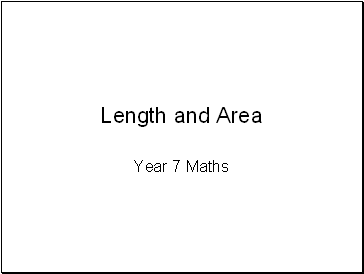Length and Area

Year 7 Maths

Slide 2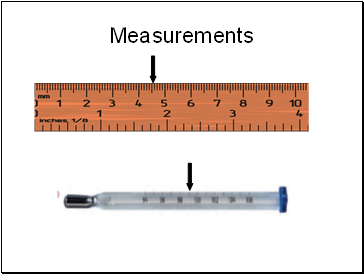## Measurements

Slide 3## Limits of accuracy

The accuracy of a measurement is how close that measurement is to the true value.

This is restricted or limited by the accuracy of the measuring instrument.

The ruler is marked in centimetres, so any length measured with it can only be given to the nearest centimetre.

Slide 4## Limit of Accuracy

For each of these measuring scales, state the size of one unit on the scale and state the limit of accuracy.

The size of one unit is 1 kg. The limits of accuracy are ±0.5 × 1 kg = ±0.5 kg.

The size of one unit is 5 mL. The limits of accuracy are ±0.5 × 5 mL = ±2.5 mL.

Slide 5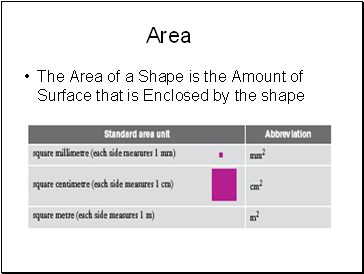## Area

The Area of a Shape is the Amount of Surface that is Enclosed by the shape

Slide 6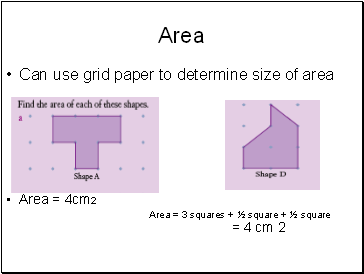Area

Can use grid paper to determine size of area

Area = 4cm2

Area = 3 squares + ½ square + ½ square = 4 cm 2

Slide 7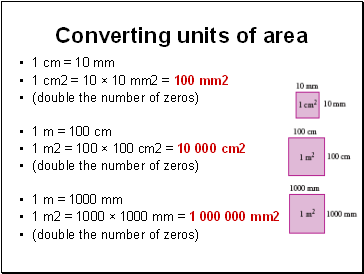## Converting units of area

1 cm = 10 mm

1 cm2 = 10 × 10 mm2 = 100 mm2

(double the number of zeros)

1 m = 100 cm

1 m2 = 100 × 100 cm2 = 10 000 cm2

(double the number of zeros)

1 m = 1000 mm

1 m2 = 1000 × 1000 mm = 1 000 000 mm2

(double the number of zeros)

Slide 8## Conversions of Units

1cm2 = 100mm2

1m2 = 10 000cm2

1m2 = 1 000 000mm2

Slide 9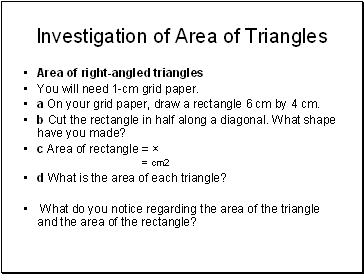## Investigation of Area of Triangles

Area of right-angled triangles

You will need 1-cm grid paper.

a On your grid paper, draw a rectangle 6 cm by 4 cm.

b Cut the rectangle in half along a diagonal. What shape have you made?

c Area of rectangle = ×

= cm2

d What is the area of each triangle?

What do you notice regarding the area of the triangle and the area of the rectangle?

Slide 10## Area of squares, rectangles and triangles

Area of square = side × side

= s × s

= s2

Area of rectangle = length × breadth

= l × b

Area of triangle = ½ × base × height

Go to page:
1  2  3